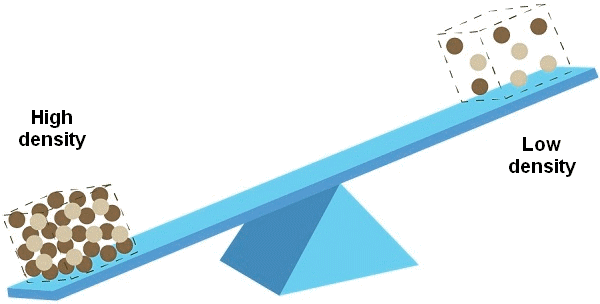# Metal Weight Calculator

Titanium
PipeInput: Millimeters Density 4.506 - g/cm3 Diameter Wall thk Length Result 0.00 kg

Disclaimer: The calculated weights are only an estimate. They are calculated based on nominal dimensions and scientifically recognized densities. In practice, the actual metal weight may differ significantly from the theoretical weight due to variations in manufacturing tolerances and compositions. This calculation form indicates the density at which the weight calculation is performed.Explore the World of Piping